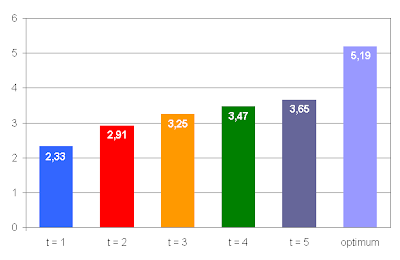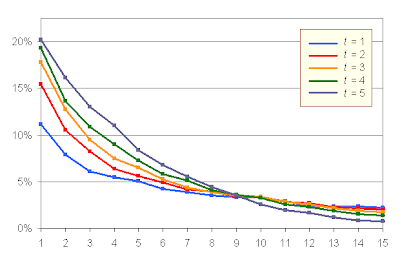## Saturday, May 24, 2008

### Making choices

In his talk The Paradox of Choice, Barry Schwartz comments on an experiment conducted around speed dating: two speed dating evenings were set up; in the first one each participant had 12 dates, and only 6 in the second event; results showed that more matches were made on the second case despite the lower number of dates. Schwartz explains the paradox as follows: each person evaluates their potential partners according to a set of traits, like sex appeal, empathy, intelligence and so on. Fully evaluating 12 candidates is a harder task than when there are only 6 candidates, so in the first case the participants decide to simplify the evaluation strategy and focus on only one personal trait, usually sex appeal, which results in worse choices than fully evaluating less candidates.

Does this explanation stand formal analysis? The hypothesis is that full evaluation of a few candidates gives better results than partial evaluation of more candidates. At first sight this is not entirely obvious, since there is a trade-off between evaluation effort per candidate and number of candidates evaluated: it well could be that sampling more candidates compensates for the poorer evaluation.

Let us build a very simple mathematical model of the situation: we have N candidates c1,...,cN to choose from, each one scoring on M different evaluation traits so that sij is the score of ci on the j-th trait. The global score of ci is then si := ∑jsij. Let us assume that the different sij are independent random variables following a normal distribution with mean 0 and variance 1. We define a selection strategy parameterized according to an integer 1 ≤ tM:

Take N/t candidates at random and choose among these one with maximum partial score s'i := si1+ ··· +sit.

So, for t = 1 the strategy consists in selecting among all the candidates one with maximum score in the first trait; for t = 2, we select half the candidates at random and choose among them based on their first two traits; for t = M, the evaluation is based on all the traits, but only for a fraction 1/M of the candidate pool. The number of trait evaluations for any value of t remains constant at (N/t candidates)·(t traits per candidate) = N, which is an expression of the expected trade-off between evaluation depth and candidate coverage. Schwartz's hypothesis states that the best strategy is t = M.

I have written a Boost-based C++ program to simulate the model for N = 60, M = 5. The figure shows the average score of the candidate chosen by the different selection strategies for t = 1,...,5, along with the the average result for the optimum choice.So, Schwartz's hypothesis is correct (at least for this scenario): the best strategy is to do full evaluation at the expense of not covering all the available candidates. We can also analyze the results of the simulation by depicting, for every t, the probability pt(n) that the strategy with that t chooses the n-th best candidate.With t = 5, the probability that we actually select the optimum candidate is 20% (obviously, since we are fully evaluating 20% of the candidate pool), while for t = 1 the probability decreases to 12%.

From this experiment, we can extract an interesting piece of advice for everyday life: when confronted with the task of selecting an option among a wide array of candidates, it is better to carefully study a small subset of candidates than to skim through them all.

From a broader perspective, the strategies we have studied are only a subset of the possible selection schemes constrained to using a fixed amount N of trait evaluations. Seen this way, there are in fact more efficient strategies than these, as we will see in a later entry.